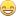## How to set constrains for this problem

Hi guys,

I am having trouble to add the new constraints for this kind of problem. The work I am doing is managing the pump and valve running over the 24 hours from 8 am to 7 am the next day.

set<num> = {8,9,10,11,...7};

set PUMP = /Pump1 Pump2 Pump3/;

var PumpStation{TIME,PUMP} Binary;

The objective function is minimized power usage.

The constraints I am working on is once the pump is on, it has to run for at least 2 hours.

The valve is the following :

var Valve{TIME} Binary;

var ValveFlow{TIME} integer;

con Flow_pb: ValveFlow[t] >= 200*Valve[t];

con Flow_lb: ValveFlow[t] <= 1000*Valve[t];

The new constraints adding in the valve is once the valve is open, the flow needs to be same for at least 4 hours.

Thank you.

1 ACCEPTED SOLUTION

Accepted Solutions

## Re: How to set constrains for this problem

I recommend declaring your time periods as follows, with the interpretation that 0 corresponds to 8am:

``````set TIME = 0..23;
``````

Then declare the following additional binary variable and linear constraints:

``````var StartPumpStation{TIME,PUMP} binary;

/* if PumpStation[t,p] = 1 and PumpStation[t-1,p] = 0 then StartPumpStation[t,p] = 1 */
con StartPumpStationCon1 {t in TIME, p in PUMP: t-1 in TIME}:
PumpStation[t,p] - PumpStation[t-1,p] <= StartPumpStation[t,p];

/* if StartPumpStation[t,p] = 1 then PumpStation[t-1,p] = 0 */
con StartPumpStationCon2 {t in TIME, p in PUMP: t-1 in TIME}:
StartPumpStation[t,p] <= 1 - PumpStation[t-1,p];

/* if StartPumpStation[t,p] = 1 then PumpStation[t,p] = PumpStation[t+1,p] = 1 */
con StartPumpStationCon3 {t in TIME, p in PUMP, t2 in t..t+1: t+1 in TIME}:
StartPumpStation[t,p] <= PumpStation[t2,p];
``````

For the valve flows, define lower and upper bounds in the declaration:

``````var ValveFlow{TIME} integer >= 0 <= 1000;
``````

Then declare another binary variable and linear constraints:

``````var StartValve {TIME} binary;

/* if Valve[t] = 1 and Valve[t-1] = 0 then StartValve[t] = 1 */
con StartValveCon1 {t in TIME: t-1 in TIME}:
Valve[t] - Valve[t-1] <= StartValve[t];

/* if StartValve[t] = 1 then Valve[t-1] = 0 */
con StartValveCon2 {t in TIME: t-1 in TIME}:
StartValve[t] <= 1 - Valve[t-1];

/* if StartValve[t] = 1 then Valve[t] = 1 */
con StartValveCon3 {t in TIME}:
StartValve[t] <= Valve[t];

/* if StartValve[t] = 1 then ValveFlow[t] = ValveFlow[t+1] = ValveFlow[t+2] = ValveFlow[t+3] */
con StartValveCon4 {t in TIME, t2 in t..t+2: t+3 in TIME}:
ValveFlow[t2] - ValveFlow[t2+1] <= (ValveFlow[t2].ub - ValveFlow[t2+1].lb) * (1 - StartValve[t]);
con StartValveCon5 {t in TIME, t2 in t..t+2: t+3 in TIME}:
ValveFlow[t2+1] - ValveFlow[t2] <= (ValveFlow[t2+1].ub - ValveFlow[t2].lb) * (1 - StartValve[t]);
``````
4 REPLIES 4

## Re: How to set constrains for this problem

Do you want the schedule to repeat every day?  I'm wondering about whether activities just before 7am need to "wrap around" to the beginning of the day.

## Re: How to set constrains for this problem

Hi there,

I only need it for one day. So starting at 8 am and ending at 7 am the next day. doesn't need to wrap it up. Thanks.

## Re: How to set constrains for this problem

I recommend declaring your time periods as follows, with the interpretation that 0 corresponds to 8am:

``````set TIME = 0..23;
``````

Then declare the following additional binary variable and linear constraints:

``````var StartPumpStation{TIME,PUMP} binary;

/* if PumpStation[t,p] = 1 and PumpStation[t-1,p] = 0 then StartPumpStation[t,p] = 1 */
con StartPumpStationCon1 {t in TIME, p in PUMP: t-1 in TIME}:
PumpStation[t,p] - PumpStation[t-1,p] <= StartPumpStation[t,p];

/* if StartPumpStation[t,p] = 1 then PumpStation[t-1,p] = 0 */
con StartPumpStationCon2 {t in TIME, p in PUMP: t-1 in TIME}:
StartPumpStation[t,p] <= 1 - PumpStation[t-1,p];

/* if StartPumpStation[t,p] = 1 then PumpStation[t,p] = PumpStation[t+1,p] = 1 */
con StartPumpStationCon3 {t in TIME, p in PUMP, t2 in t..t+1: t+1 in TIME}:
StartPumpStation[t,p] <= PumpStation[t2,p];
``````

For the valve flows, define lower and upper bounds in the declaration:

``````var ValveFlow{TIME} integer >= 0 <= 1000;
``````

Then declare another binary variable and linear constraints:

``````var StartValve {TIME} binary;

/* if Valve[t] = 1 and Valve[t-1] = 0 then StartValve[t] = 1 */
con StartValveCon1 {t in TIME: t-1 in TIME}:
Valve[t] - Valve[t-1] <= StartValve[t];

/* if StartValve[t] = 1 then Valve[t-1] = 0 */
con StartValveCon2 {t in TIME: t-1 in TIME}:
StartValve[t] <= 1 - Valve[t-1];

/* if StartValve[t] = 1 then Valve[t] = 1 */
con StartValveCon3 {t in TIME}:
StartValve[t] <= Valve[t];

/* if StartValve[t] = 1 then ValveFlow[t] = ValveFlow[t+1] = ValveFlow[t+2] = ValveFlow[t+3] */
con StartValveCon4 {t in TIME, t2 in t..t+2: t+3 in TIME}:
ValveFlow[t2] - ValveFlow[t2+1] <= (ValveFlow[t2].ub - ValveFlow[t2+1].lb) * (1 - StartValve[t]);
con StartValveCon5 {t in TIME, t2 in t..t+2: t+3 in TIME}:
ValveFlow[t2+1] - ValveFlow[t2] <= (ValveFlow[t2+1].ub - ValveFlow[t2].lb) * (1 - StartValve[t]);
``````

## Re: How to set constrains for this problem

Thank you very much RobDiscussion stats
• 4 replies
• 496 views
• 0 likes
• 2 in conversation